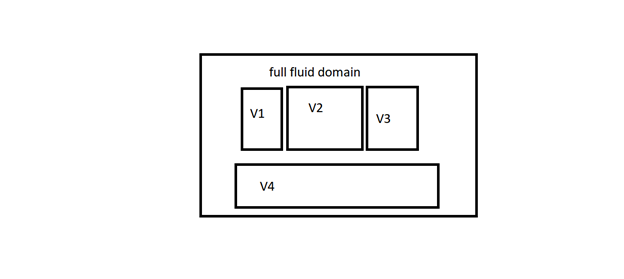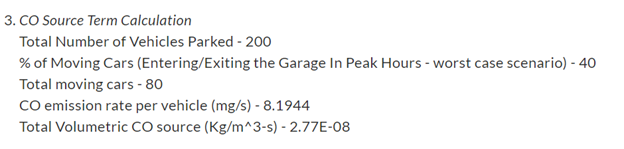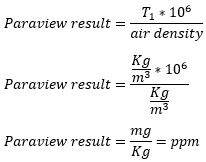# Help required while calculating passive scalar in paraview

#1

say for instance in the editorial demo

the above is the project link. he defined single passive scalar for complete volume

but my interest is to split the domain into some 3 parts and assign each passive scalar for each domain individually

however, my all zones are connected.

so, can i define for all separate zones same 1 1/m3s value

if that is the case how to calculate overall passive scalar by using the “(T1 * 2.77E-08)/(1.2041) *1000000”

the above formula mentioned as per editorial demo.in the above image, v1, v2, v3, v4 are separate zones having different number of cars in each volume in same basement. Volumes are different. So the below calculation needs to be done individually for v1, v2, v3 and v4.Now we can do it by creating each Cartesian box for v1, Cartesian box for v2 etc and assign the passive scalar i.e. 1 1/m3s for each Cartesian box separately . if I follow the above approach, then how to calculate the overall passive scalar in the entire fluid zone as per above image. I mean we cannot directly use this formula “(T1 * 2.77E-08)/(1.2041) *1000000”.

(2.77E-08 source term for the entire domain as per editorial demo) but in my case i get source term for v1, v2, v3, v4.

Any suggestions in setting up the case or while extracting results in paraview.

so i want to define different scalar values for different zones.

my question is if that is possible, when extracting results in paraview

here is my project for reference where I created different passive scalars for different zones respectively.

https://www.simscale.com/workbench/?pid=7760423999283474416&mi=spec%3A6228ef74-b2c8-450d-9b40-1726845558e5%2Cservice%3ASIMULATION%2Cstrategy%3A4&ps=geometryPrimitives%2F55

#2

@CFD-SQUAD, can someone of you maybe jump in here? Will have a look at that project later on.

Cheers guysJousef

#3

Hey rkaifi,

I believe for this case you would have to set different volumetric source fluxes for each one. The question is what would be the best approach…

I’d try the following:

• For each one of the source volumes, calculate how much CO (in Kg) would be created per second per unit of source volume. Enter this value as the passive scalar source flux for the respective volume;
• To convert it back to ppm using paraview, I believe the formula would be T1x10^6/(Air density);
• Just to doublecheck the units:Edit: I tweaked the formula a bit, since T1 is already a concentration (Kg CO/m³).

/Ric

#4

thankyou @Ricardopg. i understood celarly.